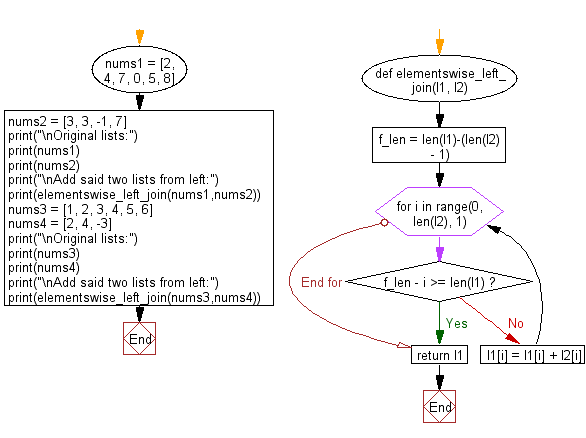﻿ Python: Add two given lists of different lengths, start from left - w3resource# Python: Add two given lists of different lengths, start from left

## Python List: Exercise - 155 with Solution

Write a Python program to add two given lists of different lengths, start from left.

Sample Solution:

Python Code:

``````def elementswise_left_join(l1, l2):
f_len = len(l1)-(len(l2) - 1)
for i in range(0, len(l2), 1):
if f_len - i >= len(l1):
break
else:
l1[i] = l1[i] + l2[i]
return l1

nums1 = [2, 4, 7, 0, 5, 8]
nums2 = [3, 3, -1, 7]
print("\nOriginal lists:")
print(nums1)
print(nums2)
print("\nAdd said two lists from left:")
print(elementswise_left_join(nums1,nums2))

nums3 = [1, 2, 3, 4, 5, 6]
nums4 = [2, 4, -3]
print("\nOriginal lists:")
print(nums3)
print(nums4)
print("\nAdd said two lists from left:")
print(elementswise_left_join(nums3,nums4))
```
```

Sample Output:

```Original lists:
[2, 4, 7, 0, 5, 8]
[3, 3, -1, 7]

Add said two lists from left:
[5, 7, 6, 7, 5, 8]

Original lists:
[1, 2, 3, 4, 5, 6]
[2, 4, -3]

Add said two lists from left:
[3, 6, 0, 4, 5, 6]
```

Flowchart:## Visualize Python code execution:

The following tool visualize what the computer is doing step-by-step as it executes the said program:

Python Code Editor:

Have another way to solve this solution? Contribute your code (and comments) through Disqus.

What is the difficulty level of this exercise?

Test your Python skills with w3resource's quiz

﻿

## Python: Tips of the Day

```print(2_000_000)
```2000000